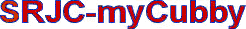SRJC - myCubbyExcellence in Education & Service • Home
home  >  myCubby  >  students  >  degree audit  > degree audit coursesNew Student Portal Student Portal Home Apply Add/Drop/Register View Grades Purchase Optional Fees Pay Fees Check myCertificate progress Check myDegree progress Schedule of Classes SRJC Schedule Planner FAQs and A&R Policies Contact Us CA Voter Registration Make a ReportCompetency in Mathematics may be demonstrated in one of the following ways:

• Achieve a satisfactory score on the Intermediate Algebra Competency Exam (ACE)
• Complete one of the following courses with a grade of 'C' or better:

 Course Description MATH 1A Calculus, First Course MATH 1B Calculus, Second Course MATH 1C Calculus, Third Course MATH 2 Calculus, Fourth Course-Differential Equations MATH 4 Discrete Mathematics MATH 9 Finite Mathematics MATH 10 Nature of Mathematics MATH 15 Elementary Statistics MATH 16 Introduction to Mathematical Analysis MATH 25 Precalculus Algebra MATH 27 Precalculus Algebra and Trigonometry MATH 58 Precalculus Trigonometry MATH 101 Mathematics for the Associate Degree MATH 154 Elementary and Intermediate Algebra For Business and STEM MATH 155 Intermediate Algebra MATH 156 Intermediate Algebra for Business and STEM Majors MATH 161 Mathematics Preparation for Statistics and Liberal Arts PSYCH 9 Introduction to Behavioral Sciences Statistics